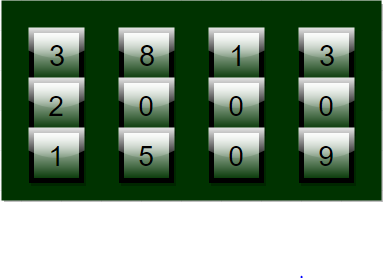# Minimum rotations to unlock a circular lock

• Difficulty Level : Easy
• Last Updated : 10 Aug, 2022

You are given a lock which is made up of n-different circular rings and each ring has 0-9 digit printed serially on it. Initially all n-rings together show a n-digit integer but there is particular code only which can open the lock. You can rotate each ring any number of time in either direction. You have to find the minimum number of rotation done on rings of lock to open the lock.Examples:

```Input : Input = 2345, Unlock code = 5432
Output : Rotations required = 8
Explanation : 1st ring is rotated thrice as 2->3->4->5
2nd ring is rotated once as 3->4
3rd ring is rotated once as 4->3
4th ring is rotated thrice as 5->4->3->2

Input : Input = 1919, Unlock code = 0000
Output : Rotations required = 4
Explanation : 1st ring is rotated once as 1->0
2nd ring is rotated once as 9->0
3rd ring is rotated once as 1->0
4th ring is rotated once as 9->0```

For a single ring we can rotate it in any of two direction forward or backward as:

• 0->1->2….->9->0
• 9->8->….0->9

But we are concerned with minimum number of rotation required so we should choose min (abs(a-b), 10-abs(a-b)) as a-b denotes the number of forward rotation and 10-abs(a-b) denotes the number of backward rotation for a ring to rotate from a to b. Further we have to find minimum number for each ring that is for each digit. So starting from right most digit we can easily the find minimum number of rotation required for each ring and end up at left most digit.

## C++

 `// CPP program for min rotation to unlock` `#include ` `using` `namespace` `std;`   `// function for min rotation` `int` `minRotation(``int` `input, ``int` `unlock_code)` `{` `    ``int` `rotation = 0;` `    ``int` `input_digit, code_digit;`   `    ``// iterate till input and unlock code become 0` `    ``while` `(input || unlock_code) {` `  `  `        ``// input and unlock last digit as reminder` `        ``input_digit = input % 10;` `        ``code_digit = unlock_code % 10;`   `        ``// find min rotation` `        ``rotation += min(``abs``(input_digit - code_digit), ` `                   ``10 - ``abs``(input_digit - code_digit));`   `        ``// update code and input` `        ``input /= 10;` `        ``unlock_code /= 10;` `    ``}`   `    ``return` `rotation;` `}`   `// driver code` `int` `main()` `{` `    ``int` `input = 28756;` `    ``int` `unlock_code = 98234;` `    ``cout << ``"Minimum Rotation = "` `        ``<< minRotation(input, unlock_code);` `    ``return` `0;` `}`

## Java

 `// Java program for min rotation to unlock` `class` `GFG` `{`   `    ``// function for min rotation` `    ``static` `int` `minRotation(``int` `input, ``int` `unlock_code)` `    ``{` `        ``int` `rotation = ``0``;` `        ``int` `input_digit, code_digit;`   `        ``// iterate till input and unlock code become 0` `        ``while` `(input>``0` `|| unlock_code>``0``) {`   `            ``// input and unlock last digit as reminder` `            ``input_digit = input % ``10``;` `            ``code_digit = unlock_code % ``10``;`   `            ``// find min rotation` `            ``rotation += Math.min(Math.abs(input_digit` `                       ``- code_digit), ``10` `- Math.abs(` `                          ``input_digit - code_digit));`   `            ``// update code and input` `            ``input /= ``10``;` `            ``unlock_code /= ``10``;` `        ``}`   `        ``return` `rotation;` `    ``}`   `    ``// driver code` `    ``public` `static` `void` `main (String[] args) {` `    ``int` `input = ``28756``;` `    ``int` `unlock_code = ``98234``;` `    ``System.out.println(``"Minimum Rotation = "``+` `                  ``minRotation(input, unlock_code));` `    ``}` `}`   `/* This code is contributed by Mr. Somesh Awasthi */`

## Python3

 `# Python3 program for min rotation to unlock`   `# function for min rotation` `def` `minRotation(``input``, unlock_code):`   `    ``rotation ``=` `0``;`   `    ``# iterate till input and unlock ` `    ``# code become 0` `    ``while` `(``input` `> ``0` `or` `unlock_code > ``0``):`   `        ``# input and unlock last digit ` `        ``# as reminder` `        ``input_digit ``=` `input` `%` `10``;` `        ``code_digit ``=` `unlock_code ``%` `10``;`   `        ``# find min rotation` `        ``rotation ``+``=` `min``(``abs``(input_digit ``-` `code_digit), ` `                    ``10` `-` `abs``(input_digit ``-` `code_digit));`   `        ``# update code and input` `        ``input` `=` `int``(``input` `/` `10``);` `        ``unlock_code ``=` `int``(unlock_code ``/` `10``);`   `    ``return` `rotation;`   `# Driver Code` `input` `=` `28756``;` `unlock_code ``=` `98234``;` `print``(``"Minimum Rotation ="``,` `       ``minRotation(``input``, unlock_code));` `    `  `# This code is contributed by mits`

## C#

 `// C# program for min rotation to unlock` `using` `System;`   `class` `GFG {`   `    ``// function for min rotation` `    ``static` `int` `minRotation(``int` `input, ` `                           ``int` `unlock_code)` `    ``{` `        ``int` `rotation = 0;` `        ``int` `input_digit, code_digit;`   `        ``// iterate till input and ` `        ``// unlock code become 0` `        ``while` `(input > 0 || ` `               ``unlock_code > 0)` `        ``{`   `            ``// input and unlock last ` `            ``// digit as reminder` `            ``input_digit = input % 10;` `            ``code_digit = unlock_code % 10;`   `            ``// find min rotation` `            ``rotation += Math.Min(Math.Abs(input_digit -` `                        ``code_digit), 10 - Math.Abs(` `                        ``input_digit - code_digit));`   `            ``// update code and input` `            ``input /= 10;` `            ``unlock_code /= 10;` `        ``}`   `        ``return` `rotation;` `    ``}`   `    ``// Driver Code` `    ``public` `static` `void` `Main () ` `    ``{` `        ``int` `input = 28756;` `        ``int` `unlock_code = 98234;` `        ``Console.Write(``"Minimum Rotation = "``+` `                         ``minRotation(input, unlock_code));` `    ``}` `}`   `// This code is contributed by Nitin Mittal`

## PHP

 ``

## Javascript

 ``

Output:

`Minimum Rotation = 12`

Time Complexity: O(log(input))

Auxiliary Space: O(1)